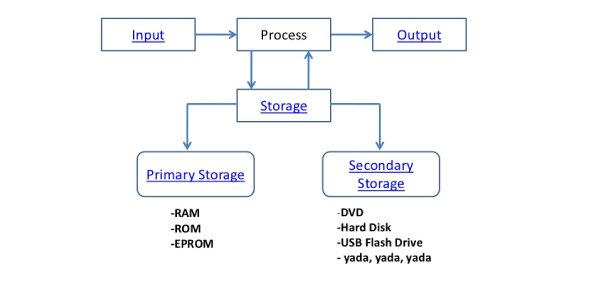# Input, Output, Processing And Storage Devices Quiz

15 Questions | Total Attempts: 11500SettingsThe basic use of computers is to input data that is processed and the output is the result of the process carried out on the data. There are a number of storage devices we can use when it comes to data and the test below is designed to test out how well you understand basic computer functions. Try playing this input, output, processing, and storage devices quiz and see just how much you know.

• 1.
Monitor/Screen is a ____________ device.
• A.

Input

• B.

Output

• C.

Processing

• D.

Storage

• E.

All of the above

• 2.
Speaker is a __________ device.
• A.

Input

• B.

Output

• C.

Processing

• D.

Storage

• E.

All of the above

• 3.
Web Cam is a __________ device.
• A.

Input

• B.

Output

• C.

Processing

• D.

Storage

• E.

All of the above

• 4.
Printer is a ___________ device.
• A.

Input

• B.

Output

• C.

Processing

• D.

Storage

• E.

All of the above

• 5.
Keyboard is a _____________ device.
• A.

Input

• B.

Output

• C.

Processing

• D.

Storage

• E.

All of the above

• 6.
Mouse is a ______________ device.
• A.

Input

• B.

Output

• C.

Processing

• D.

Storage

• E.

All of the above

• 7.
Hard Drive is a ___________ device.
• A.

Input

• B.

Output

• C.

Processing

• D.

Storage

• E.

All of the above

• 8.
CD-Rom is a _____________ device.
• A.

Input

• B.

Output

• C.

Processing

• D.

Storage

• E.

All of the above

• 9.
USB Key is a _______________ device.
• A.

Input

• B.

Output

• C.

Processing

• D.

Storage

• E.

All of the above

• 10.
Scanner is a ____________ device.
• A.

Input

• B.

Output

• C.

Processing

• D.

Storage

• E.

All of the above

• 11.
Floppy Disk is a ______________ device.
• A.

Input

• B.

Output

• C.

Processing

• D.

Storage

• E.

All of the above

• 12.
C.P.U. (Central Processing Unit) is a ____________ device.
• A.

Input

• B.

Output

• C.

Processing

• D.

Storage

• E.

All of the above

• 13.
Computer Processor is _______________ device.
• A.

Input

• B.

Output

• C.

Processing

• D.

Storage

• E.

All of the above

• 14.
CD/DVD Drive is recognized as a __________ type of device.
• A.

Storage

• B.

All of the above

• C.

Input

• D.

Output

• E.

Processing

• 15.
RAM is a ____________ type of device.
• A.

Input

• B.

Output

• C.

Processing

• D.

Storage

• E.

All of the above

Related Topics